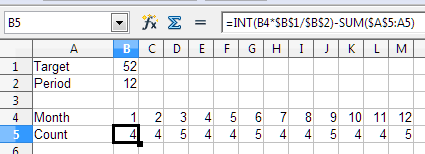How Many Houses Will I Sell Each Month?

Hypothetical problem description which will be used to do a real calculation in a client business plan.

Start with the idea that I can build and sell from one to many houses in a year, but I want to distribute the sales evenly through the year so as to stabilize my work crews. The independent variable is the total number of houses I can enter in a year. There are twelve dependent values ranging from zero to the number of houses divided by 12, calculated for each of the twelve cells representing the twelve months of the year.

Example. If I plan to build only one house, it will be sold in some month, late in the year. If I want to sell two houses, the formula will calculate a house somewhere in the latter part of each semi-annual period. If I want to sell 52 houses, I have no idea what will be in which cell, but I do know I want the sales to be evenly spread over the full twelve months.

Is there a function that I can use to divide the independent variable into integers so that the total of all the dependent integers does not exceed the independent variable? If not, can someone assist me with a formula or method of calculation?

I hope this is clear. I will try to explain anything that is not clear by adding to comments.

Thanks,
Kirk

Approach the solution to the problem as follows. To keep the construction even throughout the year, simply divide the planned number of houses by 12 months. For your example, this will be 52/12 = 4.3333. Do not be confused by the fractional part, by the end of January there will indeed be 4 full houses, completely ready for sale, and a third of a new house. Now multiply 4.3333 by the number of each of the 12 months and subtract the number of houses already sold by the end of each month.

=INT(<month>*<target>/<number_of_months>)-SUM(<prev_count>)

You will easily get 0-0-0-1-0-0-0-1-0-0-0-1 for target 3 or 4-4-5-4-4-5-4-4-5-4-4-5 for target 52I place 18 in cell C9 and the following formula in cell c24. It did not work. Where did I miss? (I only entered C\$19 rather than C\$24 as row 19 is where my text month names are and I could not figure out how to properly use the MONTH function.)

=INT(((COLUMN(C\$19)-2)*(\$C\$9)/12))-SUM(\$C\$23:\$C23)

C9? C\$19? COLUMN()? Sorry, without data it is not clearly… See example file - countHouse.ods

Maybe an image will help. My “Target” as the number of “Houses” is called in this is \$C\$9. In this case, the value is 18. I use COLUMN(C\$19)-2 as my first month is in column C and I must subtract 2 to get the column number, which gives me the value of the month since I could not figure out how to convert the text month names to their numeric equivalent. The instructions I found on the interweb did not work in my sheet. Possibly as the text is the result of a formula by the sheets creator. My SUM usage seems to match the example usage of a5

Okay. while writing this, I found my error. My corrected formula in C19 is now: =INT((COLUMN(C\$19)-2)*(\$C\$9)/12)-SUM(\$B\$21:B21) Seems I was using the wrong row id.

Oops. Thanks JohnSUN!